##### Chemistry All-in-One For Dummies (+ Chapter Quizzes Online)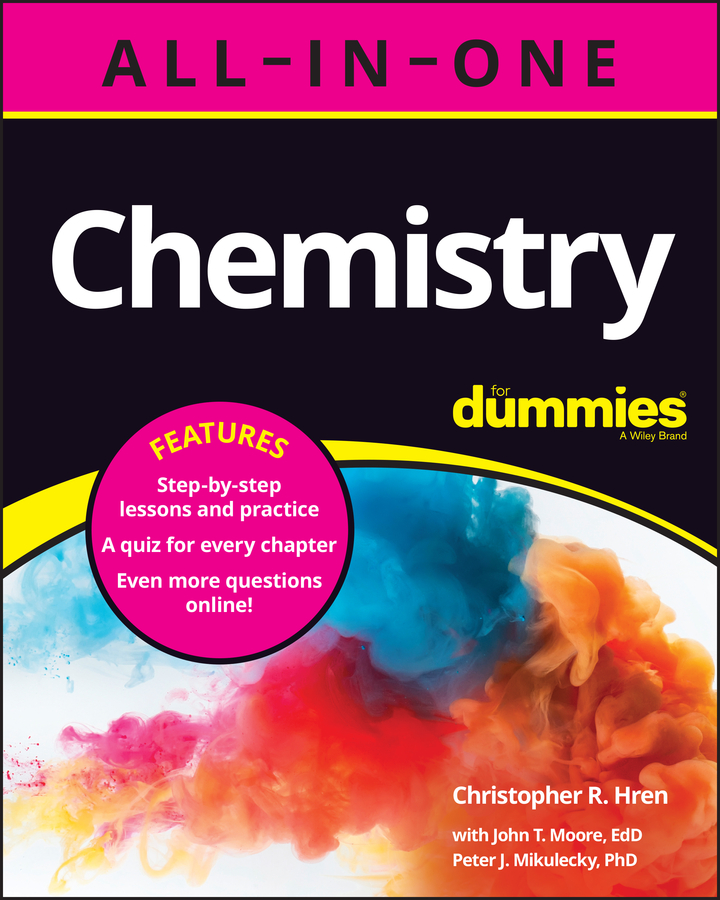When you write an equation for a chemical reaction, the two sides of the equation should balance — you need the same number of each kind of element on both sides. If you carry out a chemical reaction and carefully sum up the masses of all the reactants, and then compare the sum to the sum of the masses of all the products, you see that they’re the same.

A law in chemistry, the Law of Conservation of Mass, states, “In an ordinary chemical reaction, matter is neither created nor destroyed.” This means that you have neither gained nor lost any atoms during the reaction. They may be combined differently, but they’re still there.

A chemical equation represents the reaction. That chemical equation is used to calculate how much of each element is needed and how much of each element will be produced.

One chemical reaction is called the Haber process, a method for preparing ammonia by reacting nitrogen gas with hydrogen gas: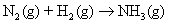This equation shows you what happens in the reaction, but it doesn’t show you how much of each element you need to produce the ammonia. To find out how much of each element you need, you have to balance the equation — make sure that the number of atoms on the left side of the equation equals the number of atoms on the right.

You know the reactants and the product for this reaction, and you can’t change them. You can’t change the compounds, and you can’t change the subscripts, because that would change the compounds.

So the only thing you can do to balance the equation is add coefficients, whole numbers in front of the compounds or elements in the equation. Coefficients tell you how many atoms or molecules you have.

For example, if you write the following, it means you have two water molecules: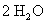Each water molecule is composed of two hydrogen atoms and one oxygen atom. So with two water molecules (represented above), you have a total of 4 hydrogen atoms and 2 oxygen atoms.

You can balance equations by using a method called balancing by inspection. You take each atom in turn and balance it by adding appropriate coefficients to one side or the other.

With that in mind, take another look at the equation for preparing ammonia:In most cases, it’s a good idea to wait until the end to balance hydrogen atoms and oxygen atoms; balance the other atoms first.

So in this example, you need to balance the nitrogen atoms first. You have 2 nitrogen atoms on the left side of the arrow (reactant side) and only 1 nitrogen atom on the right side (product side). In order to balance the nitrogen atoms, use a coefficient of 2 in front of the ammonia on the right.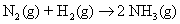Now you have 2 nitrogen atoms on the left and 2 nitrogen atoms on the right.

Next, tackle the hydrogen atoms. You have 2 hydrogen atoms on the left and 6 hydrogen atoms on the right. So put a 3 in front of the hydrogen atoms on the left, giving you: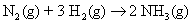That should do it. Do a check to be sure: You have 2 nitrogen atoms on the left and 2 nitrogen atoms on the right. You have 6 hydrogen atoms on the left and 6 hydrogen atoms on the right.

The equation is balanced. You can read the equation this way: 1 nitrogen molecule reacts with 3 hydrogen molecules to yield 2 ammonia molecules.

This equation would have also balanced with coefficients of 2, 6, and 4 instead of 1, 3, and 2. In fact, any multiple of 1, 3, and 2 would have balanced the equation, but chemists have agreed to always show the lowest whole-number ratio.